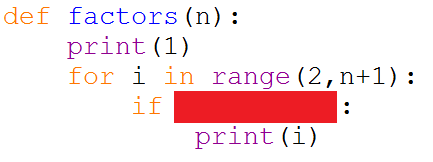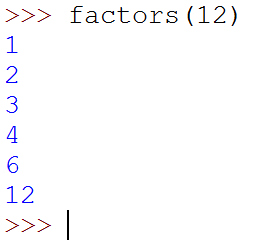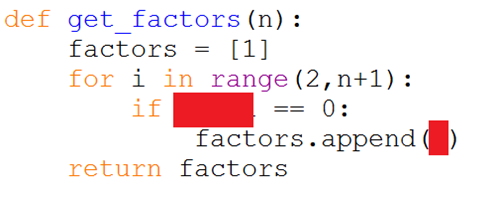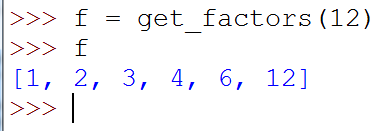# Python For GCSE Section F Exercises

Write a procedure that will print out the factors of a given number.You only have one line to work out here. Check if n is divisible by i. Test out your procedure in the shell,Writing the subroutine as a function is a little more powerful for us,With a function, we can return a list of the factors that we can use in our program later.Adapt your factors function so that it returns the proper divisors of a number. The proper divisors are the factors of the number, not including the number itself.

You only need to make a small change to the FOR loop to make this happen.

Test that your program works correctly for a range of values.

Write a function that works out the total of the proper divisors of a number.

Copy your existing proper divisors into the code window first. Your new function should start by creating a list of the proper divisors. It can do this by calling the function that you wrote for the previous task.

You can quickly work out the total of a list of integers by using the sum function. For example, total = sum(mylist)

Your function should return a single number, the total of the divisors.

Numbers which are equal to the sum of their proper divisors are called perfect numbers. Write a function that returns True if a number is a perfect number, False if it is not.

Numbers where the sum of the proper divisors is less than the number are called deficient numbers. Write a function that returns True if a number is a deficient number, False if it is not.

Numbers where the sum of the proper divisors is greater than the number are called abundant numbers. Write a function that returns True if a number is an abundant number, False if it is not.

Write a function that accepts a number and returns a string stating if the given number was deficient, perfect or abundant.

Use a for loop in the main part of the program. For the numbers 1 to 100, output the number and whether it is deficient, abundant or perfect.

Write a function to return True if a number is prime, False if it is not. Here is some pseudocode to help.

```FUNCTION Prime(n)    IF n<2 THEN       RETURN False    ELSE IF n==2 THEN       RETURN TRUE    ELSE       FOR i = 2 TO CEILING(SQROOT(n))          IF n MOD i == 0 THEN             RETURN FALSE          END IF       END FOR    END IF    RETURN True END FUNCTION```

Once you have created a function that can tell you if a number is prime, look at how to use that function in multiple contexts, including other functions that do things like,

• List primes up to a certain number.
• List the first n primes.
• List primes between two numbers.
• List primes which are also palindromic.

You can also sum these items. Write a selection of programs that make use of your prime function.

Write a Python procedure using loops, to print patterns like the following example,

*
* *
* * *
* * * *
* * * * *
* * * *
* * *
* *
*

This would be the output if you called the procedure with a 5, eg stars(5)

Write a function that accepts an integer as a parameter. Your function should return a list of the digits of that number.

There was code for this in the exercises on lists. It just needs turning into a function that returns the list.

Write a function that converts denary integers into hexadecimal strings. The following pseudocode will help.

```FUNCTION hexadecimal(n)    hexes = "0123456789ABCDEF"    answer = ""    WHILE n>0       thisplace = n MOD 16       answer = hexes[thisplace] + answer       n = n DIV 16    END WHILE    RETURN answer END FUNCTION```

Write a function that will convert a denary integer into a number in any base from 1 to 9. The principle is almost identical to the previous task. The main difference is that the number 16 will not be used, the number of the base would be. If you stick to bases from 2 to 10, you only need numeric digits.

Write a function that returns True if a string is palindromic (reads the same backwards) and False if it is not.

Use the functions that you wrote for Tasks 14 and 15. Write a new function to check if a number (n) is palindromic in a particular base (b). A single digit number is a palindrome, regardless of the number base in which it is expressed.

Write another function for number bases. This one should accept a number and return a list of the number bases in which that starting number was palindromic.

Make use of the functions that you have written for the previous tasks to produce a list of the numbers from 10 to 50. For each number, list its representation in all numbers bases from 1 to 10. Find out which numbers in the 10-50 range have the most palindromes when converted to different bases. Use your functions to explore this.